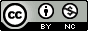## Monday, November 15, 2010

### Seminar: A New Measure of Coefficient of Determination for Regression Models

Human Genetics / Biostatistics Associate Professor (and my first statistics teacher) Dr. Chun Li will be giving a talk Wednesday on a new measure of R² for continuous, binary, ordinal, and survival outcomes. Here are the details:

Department of Biostatistics Seminar/Workshop Series

A New Measure of Coefficient of Determination for Regression Models

Chun Li, PhD

Associate Professor, Department of Biostatistics, Vanderbilt University School of Medicine

Wednesday, November 17, 1:30-2:30pm, MRBIII Conference Room 1220
http://biostat.mc.vanderbilt.edu/CLiNov17
Summary: Coefficient of determination is a measure of the goodness of fit for a model. It is best known as R² in ordinary least squares (OLS) for continuous outcomes. However, as a ratio of values on the squared outcome scale, is often not intuitive to think of. In addition, extensions of the definition to other outcome types often have unsatisfactory properties. One approach is to define a ratio of two quantities, but often such a definition does not have an underlying decomposition property that is enjoyed by . Another approach is to employ the connection of with the likelihood ratio statistic in linear regression where the residuals follow normal distributions, but for discrete outcomes, this will result in a value less than one even for a perfect model fit. For regression models, we propose a new measure of coefficient of determination as the correlation coefficient between the observed values and the fitted distributions. As a correlation coefficient, this new measure is intuitive and easy to interpret. It takes into account the variation in fitted distributions, and can be readily extended to other outcome types. For OLS, it is numerically the same as ! We present the new measure for continuous, binary, ordinal, and time-to-event outcomes.